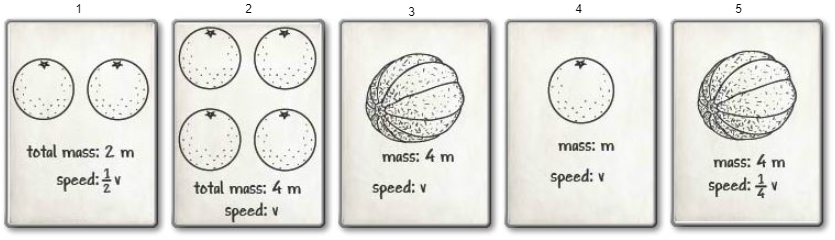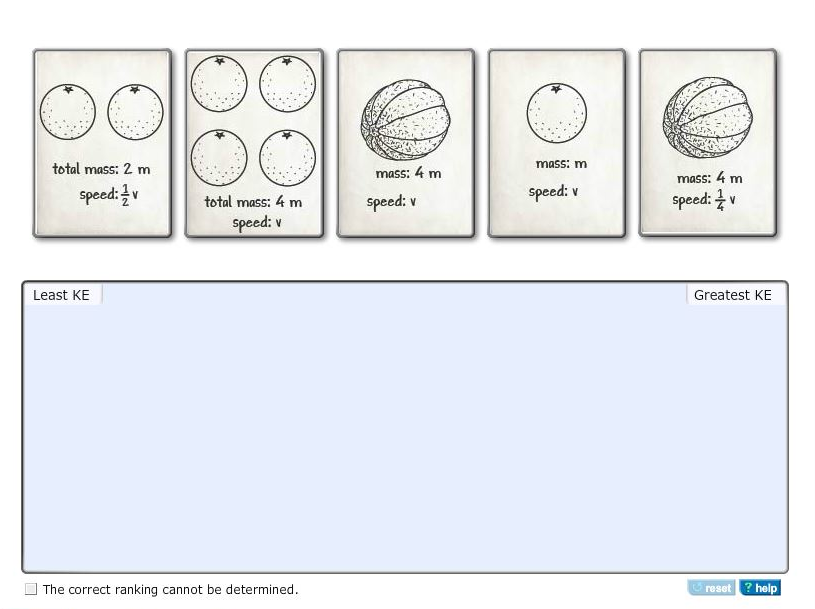# Problem: The amount of kinetic energy an object has depends on its mass and its speed.Rank the following sets of oranges and cantaloupes from least kinetic energy to greatest kinetic energy. If two sets have the same amount of kinetic energy, place one on top of the other.

###### FREE Expert Solution

Kinetic energy:

$\overline{){\mathbf{K}}{\mathbf{=}}\frac{\mathbf{1}}{\mathbf{2}}{{\mathbf{mv}}}^{{\mathbf{2}}}}$

Let's number the sets of oranges as follows:K1 = (1/2)(2m)(1/2v)2 = (1/4)mv2

86% (114 ratings)###### Problem Details

The amount of kinetic energy an object has depends on its mass and its speed.

Rank the following sets of oranges and cantaloupes from least kinetic energy to greatest kinetic energy. If two sets have the same amount of kinetic energy, place one on top of the other.